## 2. Weighted Average

It is the average of two or more groups.

Consider two groups having number of terms N1 and N2 respectively. Let the Averages of the two groups be A1 and A2 respectively.

Then the Weighted Average of the two groups is: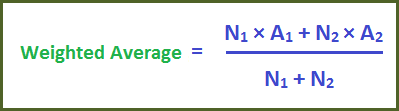Example 1:

Consider a class of 100 students with 60 boys and 40 girls. Take the average height of boys as 150 cms and the average height of girls as 130 cms. What is theaverage height of the entire class?

The average height of the whole class found from the averages (of heights) of boys and girls is called Weighted Average.

Now, recall that S = A × N

Therefore:

Sum of the heights of 60 boys = 150 × 60 = 9000 and

Sum of the heights of the 40 girls = 130 × 40 = 5200, and therefore

Grand sum of the heights of all the 100 students, including 60 boys and 40 girls is:

9000 + 5200 = 14200

Now, apply the formula for average:

Average = Sum/Num

So, A = 14200/100 = 142

This average 142 is called the weighted average of the 100 students, i.e. 60 boys and 40 girls.

Apply this same method when average (i.e. weighted average) of three or more groups is to be found.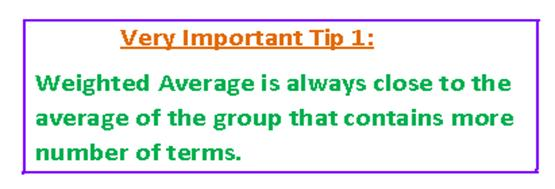In the above example, weighted average 142 is close to 150-the average of boys than to 130-the average of girls, because number of boys is more than number of girls.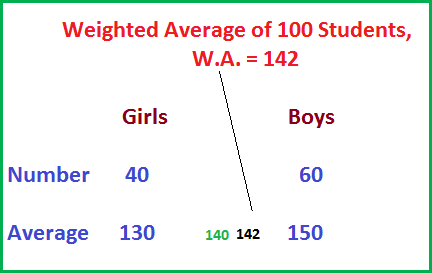Another Tip:

If number of boys and girls is same, i.e. if there are 50 boys and 50 girls, then weighted average is the average of the averages of boys and girls’ heights. i.e.

W.A. = (150 + 130)/2 = 140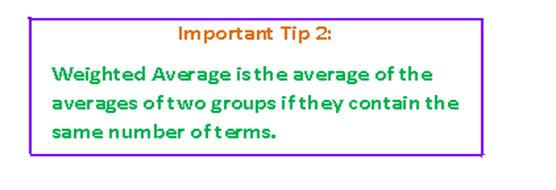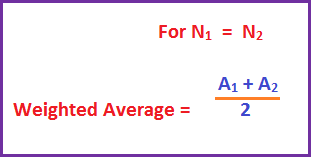Example 2:

In a class having 25 boys and 15 girls, the average age of boys is x and the average age of girls is y. Is weighted average greater than (x + y)/2, if x < y?

Solution:

Number of boys 25 is greater than number of girls 15.

Therefore, from Tip 1 above,

W.A., whatever it is, is closer to x, the average of boys than to y, the average of girls.

Now since x < y, and (x + y)/2 is the mid-value of x and y, so x < (x + y)/2

Therefore, W.A., being closer to x must be less than (x + y)/2

Hence,

W.A. < (x + y)/2

Example 3:

The average of set A = { x1 ,  x2 , x3 } is p and the average of set B =  { y1 , y2 , y3 , y4 ­, y5 } is q. What is the average of set C that contains all the elements of the two sets A and B?

Solution:

The average of set C is the W.A. of the two sets A and B.

Now, the W.A. of sets A and B is:

(p × 3 + q × 5)/ (3 + 5) = (3p + 5q)/8

Therefore, average of set C is (3p + 5q)/8

## 3. Average Speed:

Suppose you cycle to your school 2 miles away at 4 kmph and return home by the same route at 6 kmph. What is the average speed of your cycling?

Is it (4 + 6)/2 = 5 kmph?

The answer is an emphatic NO.

This is not a correct way to find average speed. Whatever average speed may be, first of all, make it clear at the outset that average speed is never found by applying average to the two speeds.

Then, what is the right answer? Here goes the solution:

Average speed = (total distance travelled)/(total time taken)

Total distance travelled is 2 + 2 = 4 miles, and

Total time taken is:

2/4 = ½ hour while going + 2/6 while coming back =

½ + 1/3 = 5/6 hours.

Therefore, average speed is

4/5/6 = 4 × 6/5 = 24/5 = 4.8 mph.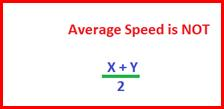Then what is Average Speed?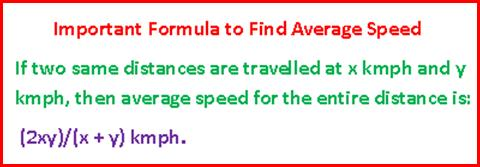Average speed = (2 × 4 × 6)/ (4 +6) = 48/10 = 4.8